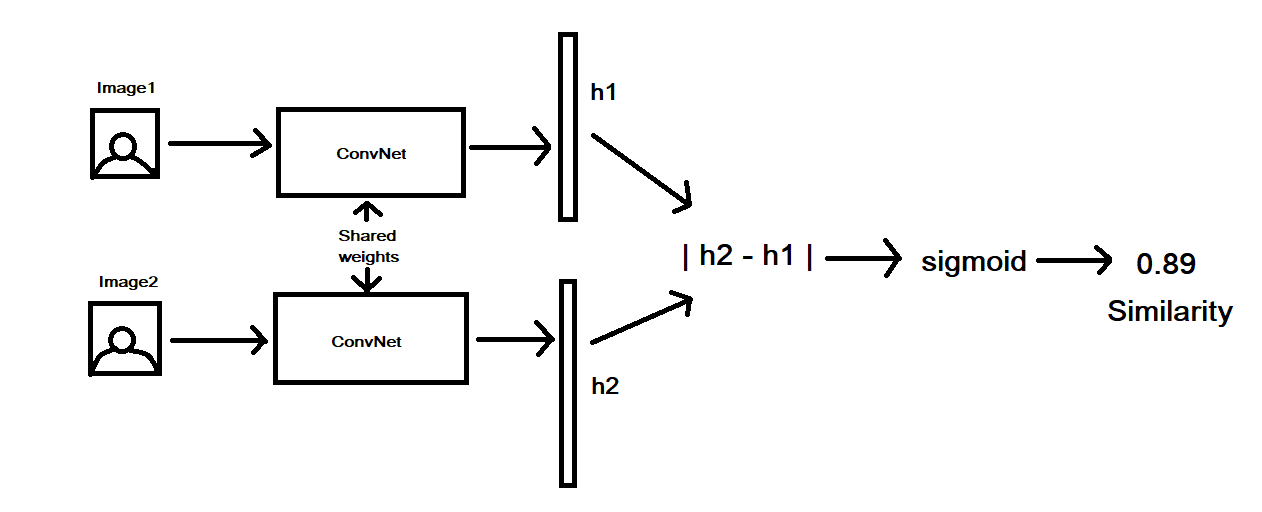# Face Recognition from Scratch using Siamese Networks and TensorFlow

### Wait. What are Siamese Networks?Heard Siamese for the first time?

The word “Siamese” means joined or connected. You probably might have heard about Siamese twins? Siamese Networks could consist of Convolutional layers as well as Dense or LSTM layers.

Since we are going to deal with images for detecting the faces, we will utilize a Convolutional Siamese Network. You can understand the architecture by this image :Siamese Network Architecture

Here’s the basic algorithm which is followed :

1. We take two images ( Image1 and Image2 ). Both the images are feed to a single Convolutional Neural Network ( CNN ).
2. The last layer of the CNN produces a fixed size vector ( embedding of the image ). Since two images are feed, we get two embeddings. ( h1 and h2 ).
3. The absolute distance between the vectors is calculated.
4. The values then pass through a sigmoid function and a similarity score is produced.

If the images are similar then the score will be closer to 1 or else it will be closer to 0.